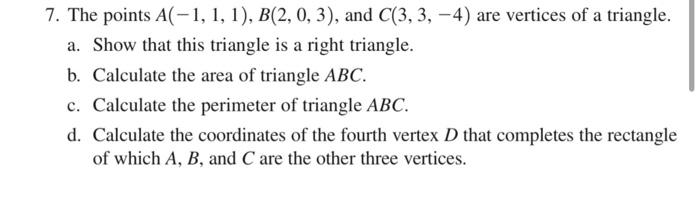Home / Expert Answers / Calculus / 7-the-points-a-1-1-1-b-2-0-3-and-c-3-3-4-are-vertices-of-a-triangle-a-show-that-this-t-pa377

# (Solved): 7. The points A(1,1,1),B(2,0,3), and C(3,3,4) are vertices of a triangle. a. Show that this t ...7. The points , and are vertices of a triangle. a. Show that this triangle is a right triangle. b. Calculate the area of triangle . c. Calculate the perimeter of triangle . d. Calculate the coordinates of the fourth vertex that completes the rectangle of which , and are the other three vertices.

We have an Answer from Expert

Solution:

Given vertices of a triangle are

Lengths of sides

(a).
Thus
Given vertices form a right-angled triangle
We have an Answer from Expert